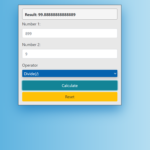## Simple JavaScript Calculator With Source Code

This Simple JavaScript calculator project is a simple project in HTML5, CSS, and JavaScript. Here, the calculator functions as that of a real-life simple calculator. This project is for solving the mathematical calculations of numbers. You can see the bas...

Type : Project

File Size : 2.1 KB# Similar Projects and Reports

#### Simple JavaScript Calculator With Source Code

This Simple JavaScript calculator project is a simple project in HTML5, CSS, and JavaScript. Here, the calculator functions as that of a real-life simple calculator. This project is for solving the mathematical calculations of numbers. You can see the bas...

#### SIMPLE CALCULATOR IN JAVASCRIPT WITH SOURCE CODE

Simple Calculator is a simple project in HTML5, CSS, and JavaScript. This project is for solving the mathematical calculations of numbers. You can see the basic features of the calculator in this project.

#### Simple JavaScript Tip Calculator With Source Code

The Simple JavaScript Tip Calculator is a simple project developed using JavaScript, CSS, and HTML. This project is an interesting project. The user can calculate the tip amount that they can get from a single bill pay. Also, the user can divide the tips ...

#### JavaScript Calculator With Source Code

This javascript calculator project is a simple project in HTML5, CSS, and JavaScript. Here, the calculator functions as that of a real-life simple calculator. This project is for solving the mathematical calculations of numbers. You can see the basic feat...

#### Simple BMI Calculator In JavaSCript with Source code

Simple BMI Calculator is a simple project developed using HTML5, CSS, and JavaScript. This project is for checking your BMI. With the BMI value, you can check whether you have a healthy weight or not. Also, you can see the basic features of this calculato...

#### Simple Tip Calculator In JavaScript With Source Code

The Simple Tip Calculator is a simple project developed using JavaScript, CSS, and HTML. This project is an interesting project. The user can calculate the tip amount that they can get from a single bill pay. Also, the user can divide the tips into number...